Updated date:

# Can you catch the dollar? - physics of free fall

Author:

Would you accept a challenge to catch a freely falling dollar? More precisely, the person who has offered a challenge holds the bill vertically (Figure 1). The centre of the bill is between other person's index finger and thumb. He or she have to catch the dollar (without moving hands down) when challenger releases the bill. Is the challenge fer or one person has a huge advantage? Our intuition may be wrong in this case. :-)

Without going into details at this moment we can immediately say that the person who accept the challenge has a small chance to win (letter we will explain). Namely, the reaction time of most people is around 0.2 second and the distance from the top of bill and the centre is at most some 10 centimetre. Freely falling body will in the first 0.2 second fall for around 20 cm. Thus, there is a little chance to catch the dollar.

Let now explain explain this trick in detail. In order to clarify the whole phenomenon, we are going to study the free fall as a motion. The intrigued fact whether the free fall depend on body mass, that dates back to Galileo Galilei's experiment in the Leaning Tower of Pisa will be explained.Fig. 1. It is not likely that with the index finger and thumb position in the middle of the bill, one can catch it. Typical reaction time is 0.2 second and in that time a body fall for around 20 cm.

## Free fall

In order to understand the free fall let analyse a movement of some freely falling body. Assume that we have a stroboscopic photograph (the one that takes photos at a constant rate) of the falling object. Say that the light flashes are taken every second. Analysing the photo we will notice one remarkable characteristic of the motion. The speed of the body increases and this increase is the same in any interval of 1 second. Of course we could choose any other interval different from a second. Thus, within the free in the same time interval the speed increases for the same value.

This rule in not characteristic only of the free fall but every motion that is subjects to this rule is called uniformly accelerated motion. The difference between initial and final speeds in a given interval very from motion to motion. Since it is the basic characteristic of such a motion we define acceleration as the ratio of speed increase to time interval in which this increase in achieved (2).

Table 1. shows data that would be obtained by such an experiment. One can see that in every tenth of second the speed increases for 0.98 m/s while in every second it increases for 9.81 m/s. The distance travelled increases progressively in the same time interval. More precisely, measuring these values one can convinces oneself that it holds relation (3).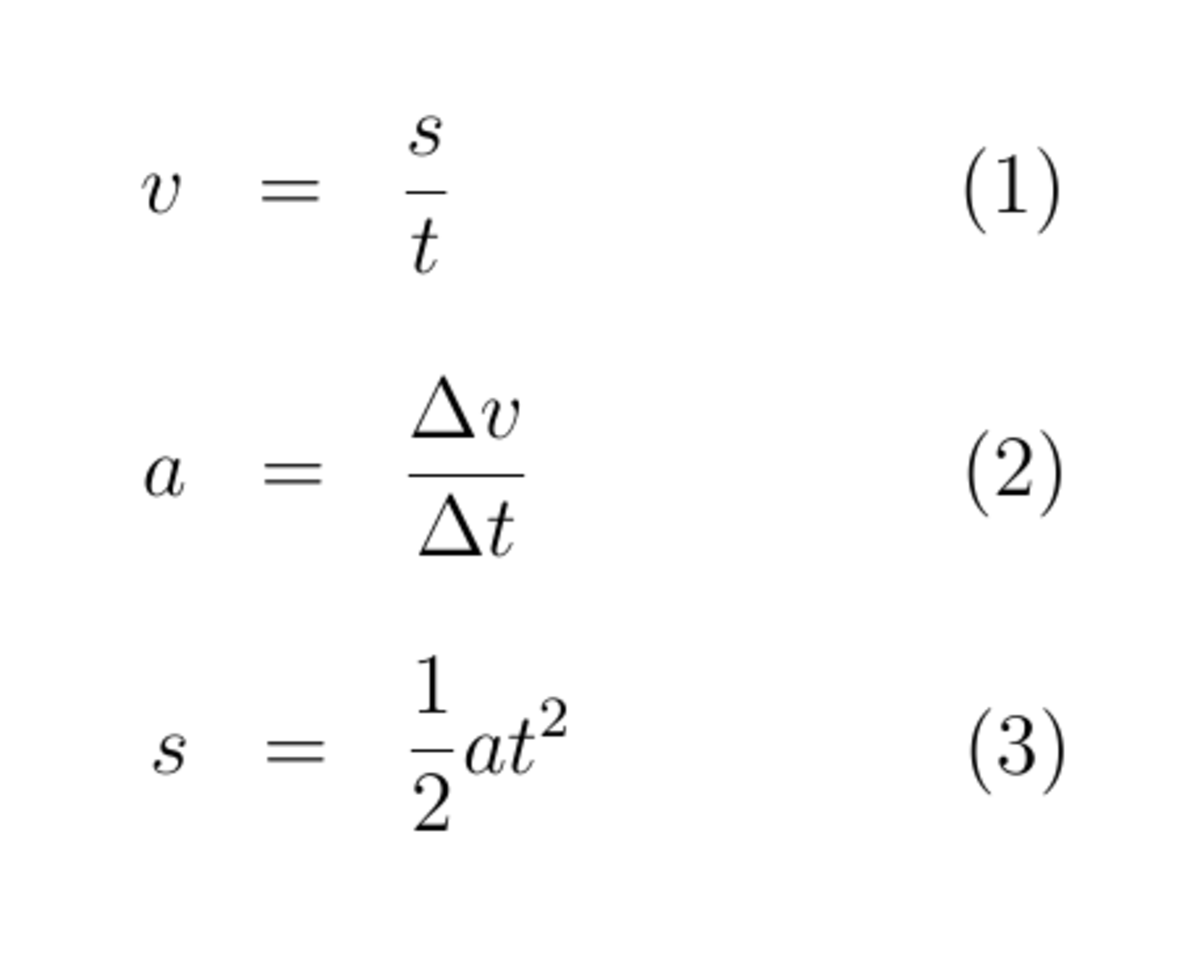Fig. 2. Relations underlying the whole kinematics. Defining relation for the speed, acceletation and relation for the distance within uniformly accelerated motion.

Table 1. Theretical results for any free falling body in the Earth's gravitational field. Real results can be different because of atmosphere.

t /sv / m/ss / mdelta v

0.1

0.98

0.05

0.2

1.96

0.20

0.98

0.3

2.94

0.44

0.98

0.5

4.91

1.23

1

9.81

4.91

2

19.62

19.62

9.81

3

29.43

44.15

9.81

4

39.24

78.48

9.81Fig. 3. Feather and apple falling in vacuum chamber. One may be surprised with this fact since our intuition is based on our experience with atmosphere.

## Uniformly accelerated motion

In the previous paragraph we were discussing the motion with constant acceleration on the case of free fall. Relations (2) and (3) worth generally for any uniformly accelerated motion and they are basic relation for such a motion. Specifically, when we talk about uniformly accelerated motion caused by the gravitational field than the acceleration is usually denoted by letter g.

In addition to the free fall (in vacuum) there is only one more system in the nature of pure uniformly accelerated motion – the frictionless incline. In both cases acceleration is caused by the gravitational interaction and don't depend on the body mass.

Presented values in the Table 1 worth for any body falling in the gravitational field with acceleration 9.81 m/s2 (which is Earth's gravitational acceleration) regardless on its mass and other characteristics. However, if experiment is performed with atmosphere than the shape of body can have significant influence on the motion (and that's why our intuition might is different) and the presented numbers would be different.

Video 1 shows the experiment performed on the Moon, as a part of scientific program of Appolo 15 mission in 1971. During a moonwalks one of astronauts test whether the hammer and feather would hit the ground at the same time. Since on the Moon there is now atmosphere the shape of body has no influence on its fall. This was a kind of verification of known theory. There is a widespread opinion that Galileo Galilei performed analogue experiments in the Leaning Tower of Pisa.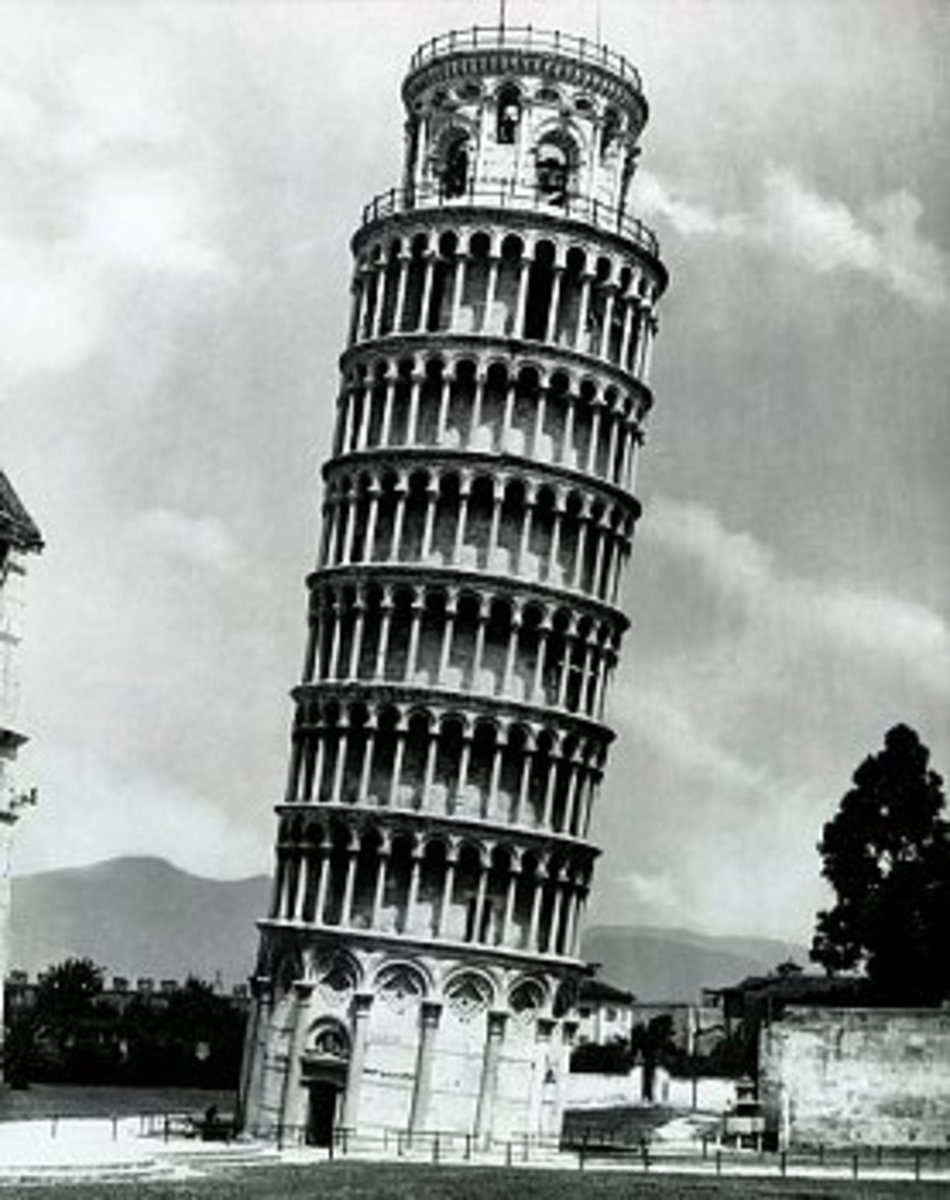Fig. 4. Leaning Tower of Pisa, where Galileo Galilei (1564-1642), an Italian physicist, mathematician and astronomer performed experiments. Galilei made a great contribution to understanding of the free fall and motion in general.

## Two elementary motion in the nature

Motions are everywhere around us, from the atomic level to the motions of astronomical objects. At first sight, some motions looks very regular while the others very irregular. While a free fall, as is explained above has constant acceleration, it is hard to notice any regularity in a typical travel by car. However, all motion in the nature, whatever be they complexity, can be decomposed to two elementary motions. These two elementary motions are uniformly linear motion and uniformly accelerated motion.

For example, a body that uniformly circle around a centre, actually perform two motion in the same time. It move with a constant speed in the tangent line whereas it move with a constant acceleration towards the centre. The net result of this two motions is uniformly circular motion. When a goalkeeper shoots a ball, its motion is again consist of two elementary motions. In the direction parallel to the ground the ball move with a constant speed whereas it has a negative acceleration vertically to the ground.

Pure elementary motions are rare in our surroundings. We already mentioned the free fall and the incline as examples for motion with constant acceleration. Close to pure uniformly linear motion will be for example hockey puck, once hit by a stick. But even in that situation, friction can't be ignored.

## Zeno's paradox: Achilles and the tortoise

It seems that for old Greek philosopher the notion of velocity was not as clear as it is nowadays. A Greek philosopher Zeno of Elea (c.490-430 BC) devised a problem about a race between Achilles and the tortoise.

In the paradox, Achilles gave the tortoise an advantage at the start, say 100 meters. Each racer run at some constant speed, Achilles fast and the the tortoise at some much smaller speed. Then, Achilles run across this 100 meters, but in meanwhile the tortoise also move at the next distance, say 10 meters. Again, Achilles run across 10 meters but the tortoise moves to the next position in that time. Thus, every time when Achilles approaches the point where the tortoise has been, it moved ahead. So, following this logic it is impossible for Achilles to overtake the tortoise. :-) What is the solution of this paradox?

## Constant speed or constant acceleration?

What is the key that differentiates if a body will move with constant speed or constant acceleration? The answer was found by Galileo Galilei and Isaac Newton.

If there is no any force acting on a body, then body will either stay at rest or move with constant speed, depending of its initial state. We say that bodies are inert (they are 'unwilling' to change its state). When some forces act on the body, the body will move with constant acceleration in the direction of net force.

These facts are the content of the first and second Newton's law of motion. The laws are against our intuition since we observe that 'for a motion a force is needed'. However, is such a reasoning the friction as a force is not taken into account.

Usually, in our environment we really need the force in order to maintain a motion but it would not be a case if the ground were frictionless.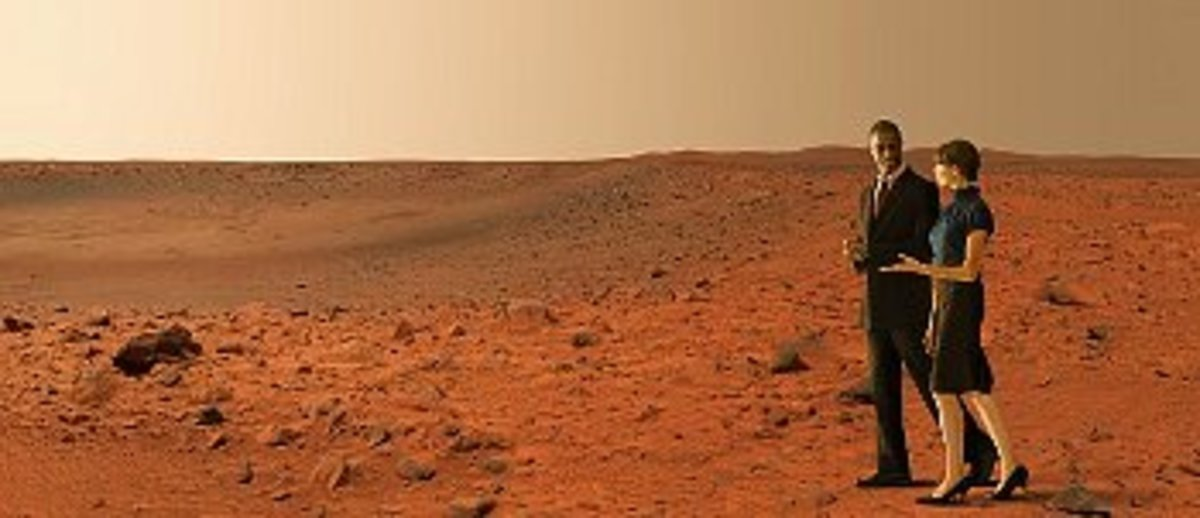Fig. 5. People on Mars. :-) Gravitational acceleration on the planet is determined by its mass. It is expected that one who on the Earth jump 2.45 m, on Mars will jump 6.12 m.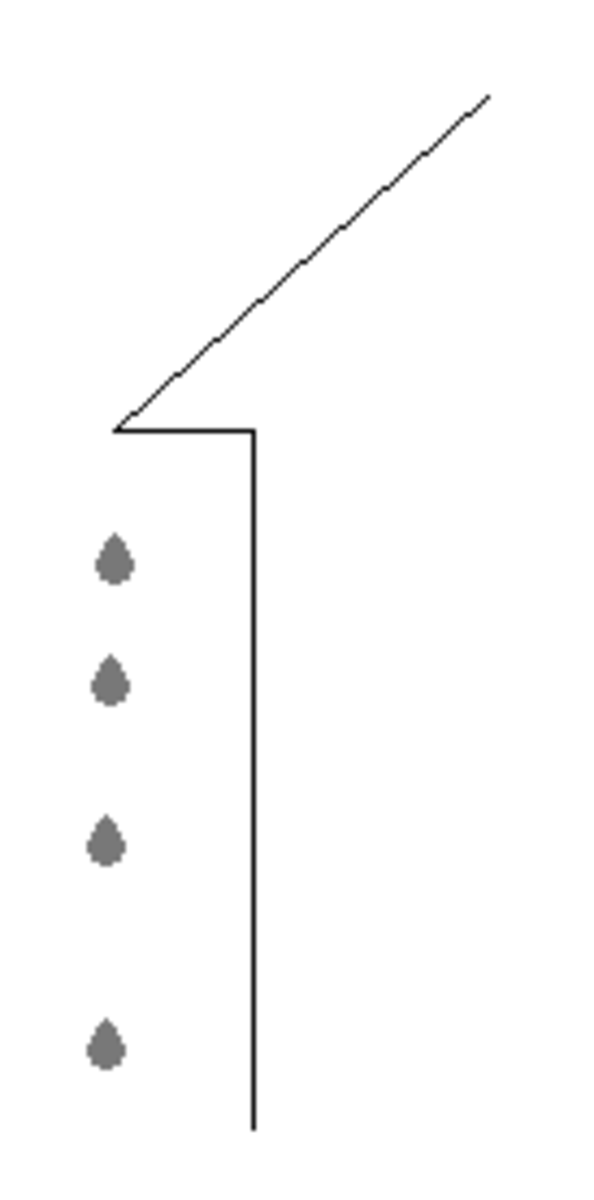Fig 6. Case study: rain droplets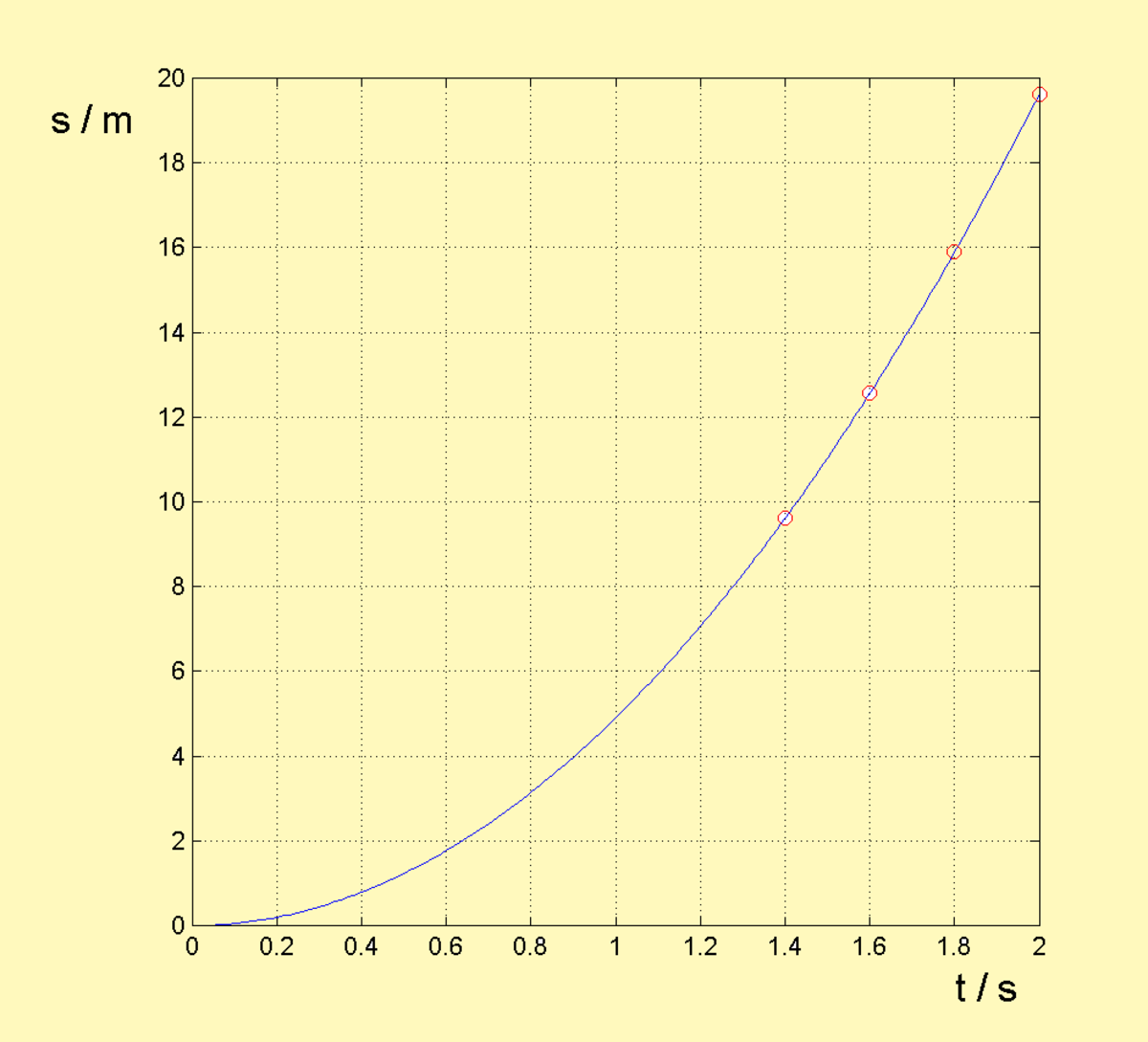Fig 7. Case study: s - t diagram of free falling droplets

## Case studies

Free falling droplets

From the roof of the skyscraper every two seconds fall a droplet of rain. What is the distance between second and third droplet 2 seconds after the first droplet fall? Air resistance let be neglected.

The solution is quite straightforward. We can easily calculate the distance that a particular droplet travel in a given time. The first droplet are falling for the t1 = 2 seconds. In that time it fall for the distance h1:

h1 = ½ * g * t12

h1 = ½ * 9.81 m/s2 * 22 s2 = 19.62 m

h2 = ½ * g * t22

h2= ½ * 9.81 m/s2 * 1.82 s2 = 15.89 m

h3 = ½ * g * t32

h3= ½ * 9.81 m/s2 * 1.62 s2 = 12.56 m

Thus, the distance between the second and third droplet 2 seconds after the first droplet starts falling will be h2 - h3= 15.89 m – 12.56 m = 3.33 m.

Divide a height on two equal times

A body is free falling from the tower of 100 meters height. Separate this height on two such a parts that for every part the body needs equal time. Air resistance let be neglected.

The plan is: firstly to calculate time for free fall from 100 meters. Than the distance that the body travel in half of this time can be calculated.

From h = ½ * g * t2 it follows t = sqrt( 2* h /g). After a short calculation it gives 4.52 s. Half of this time is 2.26 s. Now we have h2 = ½ * g * t22 = 25.05 m. Thus, in the first half of time the body travels 25.05 m while in the second half of time the body travels 75.95 m.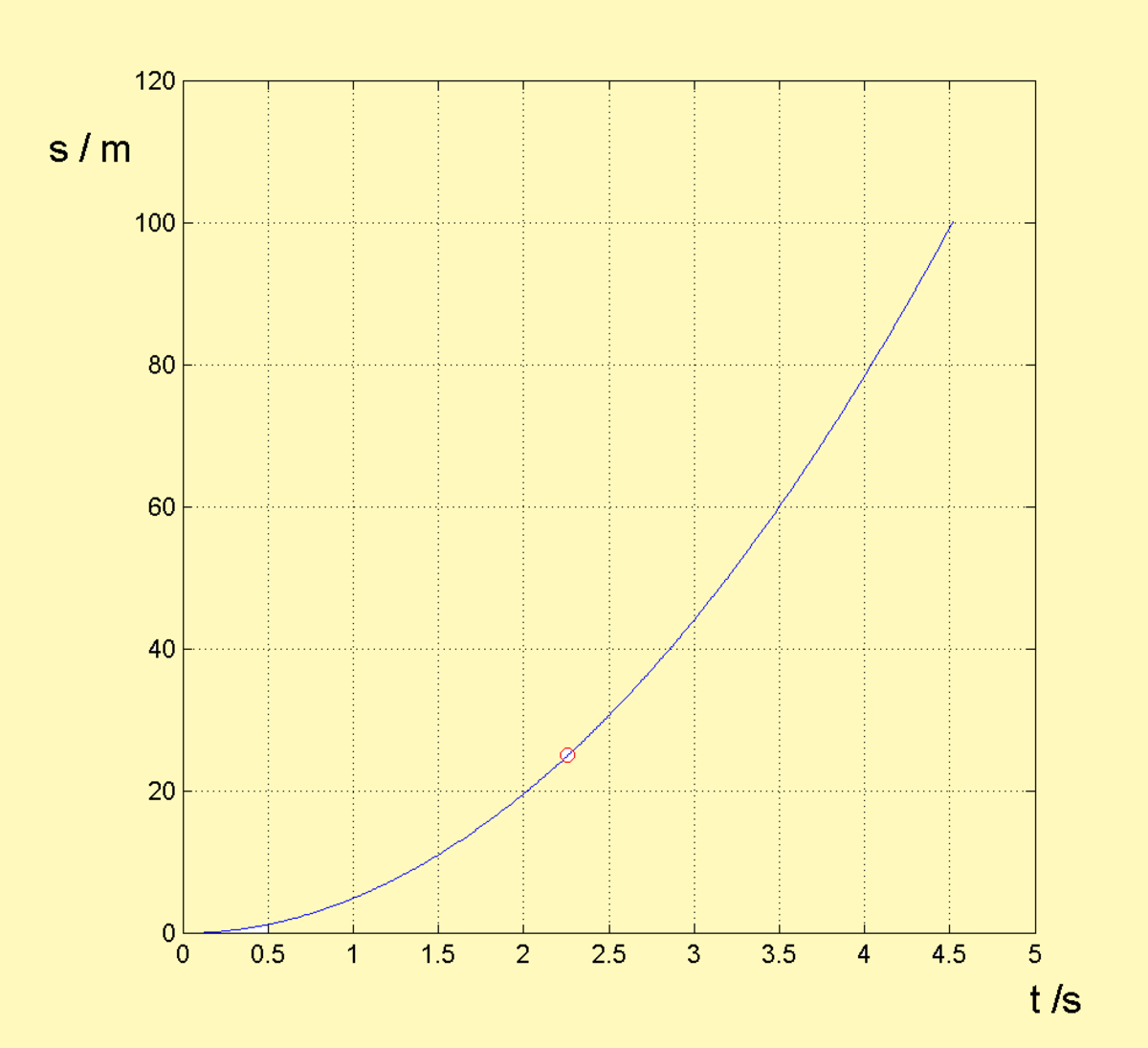Fig 8: Case study: s - t diagram of the 'divide a height on two equal times'

## A short quiz - check your knowledge!

For each question, choose the best answer. The answer key is below.

1. What does it mean that bodies are inert? Choose a true statement...
• they fall in the gravitational field
• they move with a constant speed without a force acting
• they move with a constant acceleration
• they stay at rest because of its weight
2. Who made experiments in the Leaning Tower of Pisa?
• Isaac Newton
• Galileo Galilei
• Leonardo of Pisa
• Johannes Kepler
3. Two balls glide ovet the frictionless incline, one of mass double than another. First to pass the distance will be...
• the bigger one
• the smaller one
• they will travel the distance in the same time

### Answer Key

1. they move with a constant speed without a force acting
2. Galileo Galilei
3. they will travel the distance in the same time

## Read more:

Felix Baumgartner - free fall from the edge of space